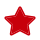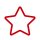Updated on 2023-03-14 GMT+08:00

# Timestamp Addition and Subtraction Operations

#### Function Name

\$timeStampCalculation(longA, StringB)

#### Parameter Description

• longA: timestamp in milliseconds.
• StringB: time difference. The value is an integer plus a letter ("d": day; "h": hour; "s": second). For example, 1d indicates that one day is added to the specified timestamp, and -1d indicates that one day is subtracted.

#### Function Description

Implements the addition and subtraction operations between parameter A of the long type and parameter B of the string type. Parameters A and B support the following types:

• Value in the format listed in Parameter Description
• Environment parameters
• Local parameters
• Other built-in functions

#### Application Scenarios

Timestamp addition and subtraction operations can be used in the following scenarios for API automation:

• Request URL
• Request body
• Checkpoint property
• if condition
• for loop interrupt condition

#### Example

• Request URL

As shown in the following figure, the value of test in the request URL is the timestamp addition and subtraction function. Parameter A in the function is 1607939485441, and parameter B is 1d.As shown in the following figure, the value of time in the request header is the timestamp addition and subtraction function. Parameter A in the function is the date-to-timestamp conversion function \$dateFormat(2020.09.11 11:00:00), and parameter B is -86400s.• Request body

As shown in the following figure, the request body uses the timestamp addition and subtraction function. Parameter A in the function is \$dateFormat(2020.09.11 11:00:00), and parameter B is 1d.• Checkpoint property

As shown in the following figure, the target value of the checkpoint property result is the timestamp addition and subtraction function. Parameter A in the function is the environment parameter time, and parameter B is -24h. For details about how to set environment parameters, see Setting Environment Parameters.• if condition

As shown in the following figure, the target value of the if condition is the timestamp addition and subtraction function. Parameter A in the function is the environment variable status, and parameter B is 1d. For details about how to set environment parameters, see Setting Environment Parameters.• for loop interrupt condition

As shown in the following figure, the target value of the for loop interrupt condition is the timestamp addition and subtraction function. Parameter A in the function is 1607939485441, and parameter B is 1d.